\$B:#>,\$N0lGU(B

\$BKhF|!":#>,\$N0lGU\$r>e\$2\$F\$\$\$^\$9!#(B
\$B:#>,0{\$`\$b\$N\$K:\$\$C\$?\$i!";29M\$K\$7\$F\$_\$F\$/\$@\$5\$\$!#(B

2019\$BG/(B9\$B7n(B

 1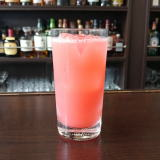(II0;2D^(B 2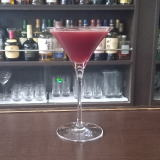(IWL^%J0SF0(B 3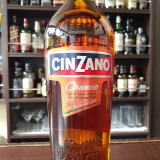(IA];^I\$B!!(I5W]A.(B 4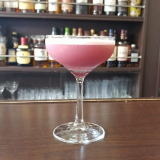(I4Y8=%53](B 5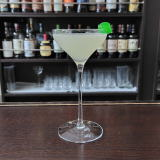(I51<=(B 6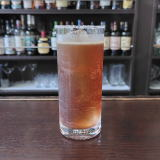(IJ^@]6^(B 7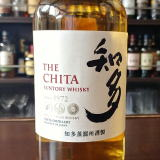\$BCNB?(B 8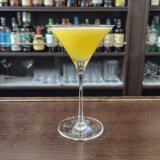(I:^0YD^%L(]6^0(B 9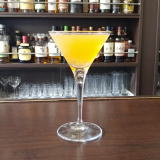(I8WX/<^(B 10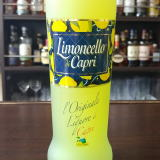(IXS]A*/[(B 11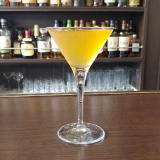(I[2TY%1]J^;@^0(B 12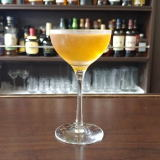(IJ^0L^D^%\2T0(B 13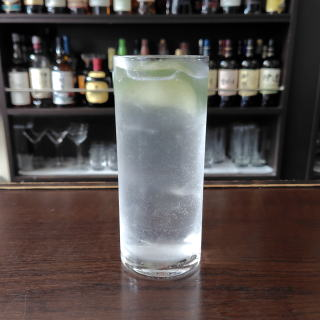(IC70W%DF/8(B 14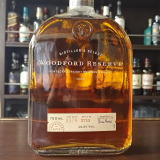(I3/D^L+0D^%X;^0L^(B 15  \$B5Y\$_(B 16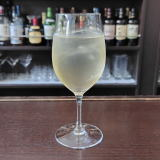(I=L_X/B'0(B 17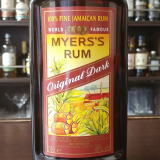(IO2T0=^%WQ(B 18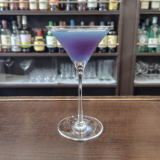(IQ0]%<,2](B 19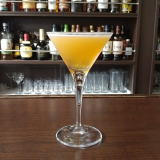(I8^X0]%Y0Q(B 20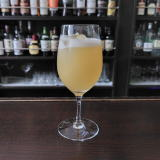(I=3(]6^0(B 21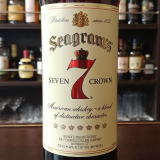(I<08^WQ\$B!!(I>L^]%8W3](B 22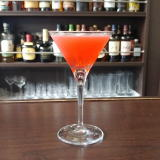(IXX1](B 23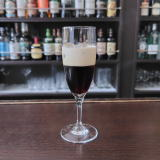(I7]8^%1YL+]?(B 24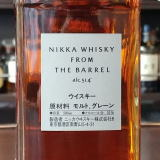(IL[Q%;^%J^ZY(B 25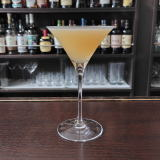(I:SD^10(B 26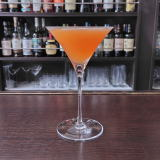(I7-0J^]%68CY(B 27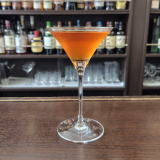(IL^XC(/<-%OC(0F(B 28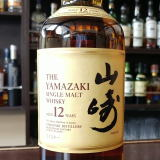\$B;3:j(B12\$BG/(B 29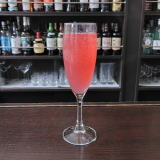(I4]<^*Y(B 30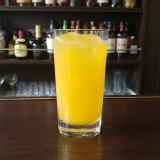(IC^(@%5Z]<^(B